# Android自定義View繪製進階-水波浪温度刻度表

theme: smartblue highlight: agate

# 波浪形温度刻度表實現

## 前言

1. 重寫測量方法，確保它是一個正方形
2. 繪製刻度
3. 繪製中心的圓與文字
4. 水波紋的動畫
5. 設置進度與動畫，一起動起來### 1、onMeasure重新測量

widthMeasureSpec ，heightMeasureSpec 並不是真正的寬高，看名字就知道，它只是寬高測量的規格，我們通過 MeasureSpec 的一些靜態方法，通過它們拿到一些信息。

static int getMode(int measureSpec):根據提供的測量值(規格)提取模式(上述三個模式之一)

static int getSize(int measureSpec):根據提供的測量值(規格)提取大小值(這個大小也就是我們通常所説的大小)

static int makeMeasureSpec(int size,int mode):根據提供的大小值和模式創建一個測量值(規格)

`onMeasure` 的最終設置確定寬度的測量有兩種方式， 1. setMeasuredDimension(width, height) 2. super.onMeasure(widthMeasureSpec,heightMeasureSpec）

``````    //獲取控件的寬度，高度
int widthMode = MeasureSpec.getMode(widthMeasureSpec);
int newWidthMeasureSpec = widthMeasureSpec;

//如果沒有指定寬度，默認給200寬度
if (widthMode != MeasureSpec.EXACTLY) {
newWidthMeasureSpec = MeasureSpec.makeMeasureSpec(200, MeasureSpec.EXACTLY);
}

//獲取到最新的寬度

//我們要的是矩形，不管高度是多高，讓它總是和寬度一致
int height = width;

centerPosition.x = width / 2;
centerPosition.y = height / 2;
mRectF.set(0f, 0f, width, height);

//最後設置生效-下面兩種方式都可以
// setMeasuredDimension(width, height);

super.onMeasure(
MeasureSpec.makeMeasureSpec(width, MeasureSpec.EXACTLY),
MeasureSpec.makeMeasureSpec(height, MeasureSpec.EXACTLY)
);

}
``````

```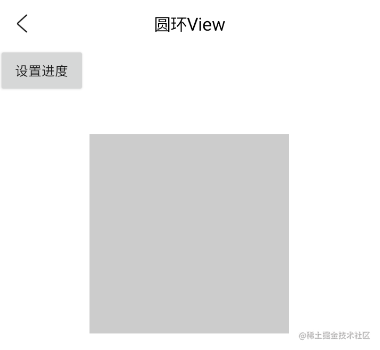### 2、繪製刻度

``````    //畫圓環
canvas.drawArc(
mRectF.left + 2f, mRectF.top + 2f, mRectF.right - 2f, mRectF.bottom - 2f,
mStartAngle, mSweepAngle, false, mDegreeCirPaint
);
}
``````

```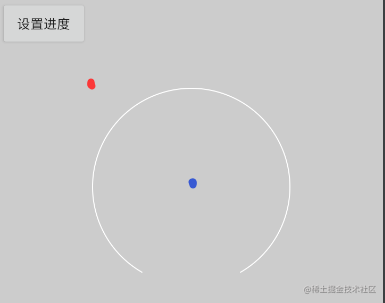``````private float mStartAngle = 120f;  // 圓弧的起始角度
private float mSweepAngle = 300f; //繪製的起始角度和滑過角度(繪製300度)
private float mTargetAngle = 300f;  //刻度的角度(根據此計算需要繪製有色的進度)

private void drawDegreeLine(Canvas canvas) {
//先保存
canvas.save();

// 移動畫布
// 旋轉座標系,需要確定旋轉角度
canvas.rotate(30);

// 每次旋轉的角度
float rotateAngle = mSweepAngle / 100;
// 累計疊加的角度
float currentAngle = 0;
for (int i = 0; i <= 100; i++) {

if (currentAngle <= mTargetAngle && mTargetAngle != 0) {
// 計算累計劃過的刻度百分比
float percent = currentAngle / mSweepAngle;

//動態的設置顏色
mDegreelinePaint.setColor(evaluateColor(percent, Color.GREEN, Color.RED));

// 畫過的角度進行疊加
currentAngle += rotateAngle;

} else {
mDegreelinePaint.setColor(Color.WHITE);
}

//畫完一個刻度就要旋轉移動位置
canvas.rotate(rotateAngle);
}

//再恢復
canvas.restore();

}
``````

```

### 3. 設置刻度動畫

```java

``````//動畫狀態
private boolean isAnimRunning;
// 手動實現越來越慢的效果
private int[] slow = {10, 10, 10, 8, 8, 8, 6, 6, 6, 6, 4, 4, 4, 4, 2};
// 動畫的下標
private int goIndex = 0;

//設置温度，入口的開始
public void setupTemperature(float temperature) {
mCurPercent = 0f;
totalAngle = (temperature / 100) * mSweepAngle;
targetAngle = 0f;
mCurPercent = 0f;
mCurTemperature = "0.0";
mWaveUpValue = 0;

startTimerAnim();
}

//使用定時任務做動畫
private void startTimerAnim() {

if (isAnimRunning) {
return;
}

mAnimTimer = new Timer();

@Override
public void run() {

isAnimRunning = true;
targetAngle += slow[goIndex];
goIndex++;
if (goIndex == slow.length) {
goIndex--;
}
if (targetAngle >= totalAngle) {
targetAngle = totalAngle;
isAnimRunning = false;
mAnimTimer.cancel();
}

// 計算的温度
mCurPercent = targetAngle / mSweepAngle;
mCurTemperature = mDecimalFormat.format(mCurPercent * 100);

// 水波紋的高度
mWaveUpValue = (int) (mCurPercent * (mSmallRadius * 2));

postInvalidate();
}
}, 250, 30);

}
``````

```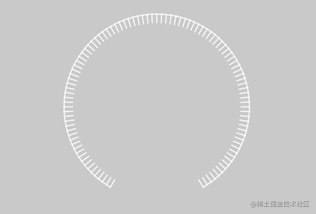### 4. 繪製中心的圓與文字

```java ...
// 計算的温度 mCurPercent = targetAngle / mSweepAngle; mCurTemperature = mDecimalFormat.format(mCurPercent * 100);

``````postInvalidate();

...
``````

```

```java private float mSmallRadius = 0f; private Paint mTextPaint; private Paint mSmallCirclePaint; private float mCurPercent = 0f; //進度 private String mCurTemperature = "0.0"; private DecimalFormat mDecimalFormat;

``````private void init() {
...

mTextPaint = new Paint();
mTextPaint.setAntiAlias(true);
mTextPaint.setTextAlign(Paint.Align.CENTER);
mTextPaint.setColor(Color.WHITE);

mSmallCirclePaint = new Paint();
}

@Override
protected void onDraw(Canvas canvas) {
super.onDraw(canvas);

...

//畫小圓
drawSmallCircle(canvas, evaluateColor(mCurPercent, Color.GREEN, Color.RED));

//畫中心的圓與文本
drawTemperatureText(canvas);

}
``````

```

``````private void drawTemperatureText(Canvas canvas) {

//提示文字
canvas.drawText("當前温度", centerPosition.x, centerPosition.y - mSmallRadius / 2f, mTextPaint);

//温度文字
canvas.drawText(mCurTemperature, centerPosition.x, centerPosition.y + mSmallRadius / 4f, mTextPaint);

//繪製單位
canvas.drawText("°C", centerPosition.x + (mSmallRadius / 1.5f), centerPosition.y, mTextPaint);

}
``````

```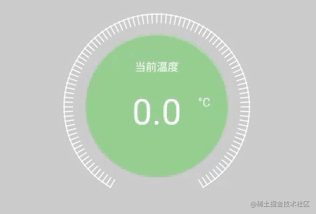### 5. 水波紋動畫

x軸其實就是我們的控件寬度，我們先用一個數組保存起來

```java private float[] mFirstWaterLine; private float[] mSecondWaterLine;

`````` @Override
protected void onMeasure(int widthMeasureSpec, int heightMeasureSpec) {

//獲取控件的寬度，高度
int widthMode = MeasureSpec.getMode(widthMeasureSpec);
int newWidthMeasureSpec = widthMeasureSpec;

//如果沒有指定寬度，默認給200寬度
if (widthMode != MeasureSpec.EXACTLY) {
newWidthMeasureSpec = MeasureSpec.makeMeasureSpec(200, MeasureSpec.EXACTLY);
}

//獲取到最新的寬度

//我們要的是矩形，不管高度是多高，讓它總是和寬度一致
int height = width;

mFirstWaterLine = new float[width];
mSecondWaterLine = new float[width];

super.onMeasure(
MeasureSpec.makeMeasureSpec(width, MeasureSpec.EXACTLY),
MeasureSpec.makeMeasureSpec(height, MeasureSpec.EXACTLY)
);

}
``````

```

``````//使用定時任務做動畫
private void startTimerAnim() {

if (isAnimRunning) {
return;
}
mAnimTimer = new Timer();

@Override
public void run() {

...

// 計算的温度
mCurPercent = targetAngle / mSweepAngle;
mCurTemperature = mDecimalFormat.format(mCurPercent * 100);

// 水波紋的高度
mWaveUpValue = (int) (mCurPercent * (mSmallRadius * 2));

postInvalidate();
}
}, 250, 30);

}

public void moveWaterLine() {
mWaveTimer = new Timer();

@Override
public void run() {
mWaveMoveValue += 1;
if (mWaveMoveValue == 100) {
mWaveMoveValue = 1;
}
postInvalidate();
}
}, 500, 200);
}
``````

```

```java /* * 繪製水波 / private void drawWaterWave(Canvas canvas, int color) {

``````    int len = (int) mRectF.right;

// 將週期定為view總寬度
float mCycleFactorW = (float) (2 * Math.PI / len);

// 得到第一條波的峯值
for (int i = 0; i < len; i++) {
mFirstWaterLine[i] = (float) (10 * Math.sin(mCycleFactorW * i + mWaveMoveValue) - mWaveUpValue);
}
// 得到第一條波的峯值
for (int i = 0; i < len; i++) {
mSecondWaterLine[i] = (float) (15 * Math.sin(mCycleFactorW * i + mWaveMoveValue + 10) - mWaveUpValue);
}

canvas.save();

// 裁剪成圓形區域
Path path = new Path();
canvas.clipPath(path);
path.reset();

// 將座標系移到底部

mSmallCirclePaint.setColor(color);

for (int i = 0; i < len; i++) {
canvas.drawLine(i, mFirstWaterLine[i], i, len, mSmallCirclePaint);
}
for (int i = 0; i < len; i++) {
canvas.drawLine(i, mSecondWaterLine[i], i, len, mSmallCirclePaint);
}

canvas.restore();

}
``````

```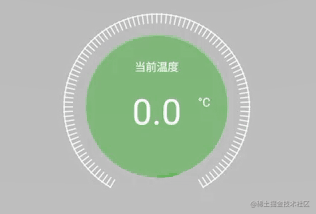```java @Override protected void onDetachedFromWindow() { super.onDetachedFromWindow(); if (mWaveTimer != null) { mWaveTimer.cancel(); } if (mAnimTimer != null && isAnimRunning) { mAnimTimer.cancel(); } }```

```kotlin findViewById(R.id.set_progress).click {

``````       val temperatureView = findViewById<TemperatureView>(R.id.temperature_view)
temperatureView .setupTemperature(70f)
}
``````

```

## 後記

Ok,這一期就此完結。